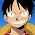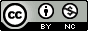## Thursday, February 24, 2011

### Split a Data Frame into Testing and Training Sets in R

I recently analyzed some data trying to find a model that would explain body fat distribution as predicted by several blood biomarkers. I had more predictors than samples (p>n), and I didn't have a clue which variables, interactions, or quadratic terms made biological sense to put into a model.

I then turned to a few data mining procedures that I learned about during grad school but never really used (LASSO, Random Forest, support vector machines, etc). So far, Random Forest is working unbelievably well. The boostrapping and aggregation ("bagging," i.e. the random component of Random Forest) avoids overfitting so well that I'm able to explain about 80% of the variation in an unseen sample using a model derived from only 30 training samples. (This paper offers the best explanation of Random Forest I've come across).

While doing this I needed to write an R function to split up a dataset into training and testing sets so I could train models on one half and test them on unseen data. I'm sure a function already exists to do something similar, but it was trivial enough to write a function to do it myself.

This function takes a data frame and returns two dataframes (as a list), one called trainset, one called testset.

```splitdf <- function(dataframe, seed=NULL) {
if (!is.null(seed)) set.seed(seed)
index <- 1:nrow(dataframe)
trainindex <- sample(index, trunc(length(index)/2))
trainset <- dataframe[trainindex, ]
testset <- dataframe[-trainindex, ]
list(trainset=trainset,testset=testset)
}
```

In R, you can generally fit a model doing something like this:

```mymodel <- method(y~x, data=mydata)
```
...and then predict the outcome for new data using the generic predict function:

```predvals <- predict(mymodel, newdataframe)
```

Here's some R code that uses the built in iris data, splits the dataset into training and testing sets, and develops a model to predict sepal length based on every other variable in the dataset using Random Forest.

*Edit 2011-02-25* Thanks for all the comments. Clearly the split() function does something very similar to this, and the createDataPartition() function in the caret package does this too.

1.Thanks, very much for an interesting post. I have played around with random forest analysis in Matlab and have now made the switch to R for data analysis. I have copied your script for future reference. I really like that random forests analysis makes it easy to tease out the contribution of individual variables to the overall classification. Great for triming a long list of biomarkers down to the relevant ones. In terms of explaination I like the chapter in "The Elements of Statistical Learning by Hastie Tibshirani and Friedman".

2.Cool work, but split() does exactly this. unsplit() is quite nice too.

3.Take a look at the caret package. It does this and a lot more.

4.Have a look at package caret. It will do the job (including the selection of training/test sets).

5.This comment has been removed by the author.

6.Hi
I don't know why you need to split data into training and test sets. random Forest produces its own 'out of bag' error estimate by growing trees on two thirds and testing on one third of the data (or thereabouts) whilst running. So you should use the whole dataset.

For the other methods there are R packages that handle cross validation and feature selection automatically like 'ipred' and 'MLInterfaces'. Cross validation is far superior to a single train and test set. Indeed you should only choose a single train and test set if they were drawn separately i.e. a model based on patients from a clinical trial is tested on patients from a new hospital.

7.I agree with Jan.

Max Kuhn's caret package has many more desirable features to compose training and test sets.

+ he replies promptly if you have questions :-)

8.You may also want to take a look at Friedman's stochastic gradient boosting (package is available in R). It's been shown to outperform Random Forest.

I will also agree with another comment on test set not neccesarily needed. If you do the cross-validation, it gives quite accurate prediction of the error.

9.You can use your test set with two different purposes:

1) You use it as a completely independent test set in order to be able to have an estimate about how well your model will behave in completely unseen data.

In this case you should never use your test set to choose your model. That means that you shouldn't repeat an experiment because you are not satisfied with the result in the test set.

2) You use it as a validation set to choose your best model.

In this case you might have more confidence that you are choosing the right model, but the result you get at the end is biased and you can't use it as an estimate of the error of your model in unseen data.

Finally, I also prefer k-fold cross-validation to choose the best algorithms and adjust parameters. With this approach, the result cannot be used as an estimate of the error in unseen data, but the methodology is very good to compare performances of the different algorithms and parameters.

10.Thanks a lot! It also works if I want 1/3 in test and 2/3 in training.

1.how did you split it to 1/3 test and 2/3 training?

2.Modify this line:

trainindex <- sample(index, trunc(length(index)/2))

11.Thanks for sharing, I will bookmark and be back again

Testing Training with Live Project

12.Thanks for this, I found it very useful. If I'm not mistaken, the seed setting in your function offers something that neither split nor createDatapartition has, the ability to reconstruct your sets later... is this correct?

13.Yep, that's exactly what I'm doing with the set seed.

14.Thank you very much! I'm modifying your function so I can input whatever size of training data set I want. Don't worry, I'll cite you in my assignment :-)

15.Thank you for this function, helped me a lot on my assignment. (Also, duly cited :) )

16.Hello everyone, could anyone help me how to calculate the confusion matrix?

17.This comment has been removed by the author.

18.This comment has been removed by the author.

19.Thanks for sharing! that was quite useful! But consider a dataset in which you have subjects divided into 2 groups(patients and control subjects) and you wish to split the main dataset in training and test set (as you did) WHILE maintaining the proportions of subjects in group1 and group2 in each set at the same ratio as in the complete data set. How would you do that?

20.Thanks for Sharing! It's very helpful

21.This post was helpful! I used your function and modified it to control the size of the respective train and test sets. I didn't know about the createDataPartition. I'll try to incorporate this into workflow for some cross validation I need to do.

The modification I made was to add the desired size of the train, with the complement still going into the test set.

splitDataFrame <- function(dataframe, seed = NULL, n = trainSize) {
if (!is.null(seed)) set.seed(seed)
index <- 1:nrow(dataframe)
trainindex <- sample(index, n)
trainset <- dataframe[trainindex, ]
testset <- dataframe[-trainindex, ]
list(trainset = trainset, testset = testset)
}

With example of calling the function with desired size for the train set, 72% of records to train set with 28% to test:

dataList <- splitDataFrame(workingData, NULL, round(nrow(workingData) * 0.72))
train <- dataList\$trainset
test <- dataList\$testset

22.works well thanks

23.hello everyone, how can i get the coefficients for my model that i have generated with this function. the normal, summary(model) or coefficients(model) are not giving me desired results. I want to get the intercept and the predictors intercepts

24.hello everyone, please i would like to split the data i am working on into 70% trainset and 30% trainset randomly 150 times! with each time having different combination of samples. Kindly help on this.

25.Hello everyone. Great job for this function, congratulations and thank you all. However, the test subset has (follows) the samen order than within full (i.e. original) data set (-> dataframe). Following I show a little modification of the function to sample the test set.

plitdf <- function(dataframe, seed=NULL) {
if (!is.null(seed)) set.seed(seed)
index_train <- 1:nrow(dataframe)
trainindex <- sample(index_train, trunc(length(index_train)/2), replace = FALSE)
trainset <- dataframe[trainindex, ]
testset_0 <- dataframe[-trainindex, ] # This subset follow the same order than original dataframe

index_test <- 1:nrow(testset_0)
testindex <- sample(index_test, trunc(length(index_test)), replace = FALSE)
testset <- testset_0[testindex, ]

# View(testset_0);View(testset); # for debugging

list(trainset=trainset,testset=testset)
}